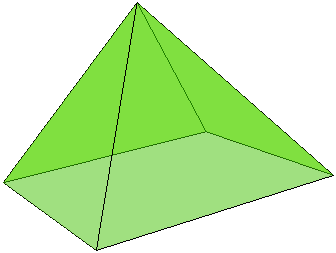# Volumes of Two Pyramids

Stuart Anderson has posed the question of comparing the volumes V of a tetrahedron (3-pyramid) and W of a square pyramid (4-pyramid), with all 14 edges they have between them equal. What do you think is the relationship between V and W?SolutionWe shall show that W = 2V.

### Solution 1

Assuming that the length of the common edges is 1, V = 2/12, whereas W = 2/6, so that 2V = W. Calculations are not difficult. The volume of a pyramid equals 1/3 of the altitude times the area of the base.

The altitude of a square pyramid is the altitude in an isosceles triangle with sides 3/2, 3/2, 1, which is 2/2. W = 1/3×2/2×1 = 2/6.

The altitude of a regular tetrahedron, is the altitude to the side of the isosceles triangle with sides 3/2, 3/2, 1, which is 6/3. V = 1/3×6/3×3/4, the latter factor being the area of the unit equilateral triangle. Thus V = 2/12.

### Solution 2

By Stuart Anderson.

Let the bases of the pyramids tile the plane with squares, draw a second plane through the apices of the pyramids, and let a second set of inverted pyramids tile this plane with their bases, the corners of each base coinciding with the apices of the upright pyramids. Since the volume of a pyramid is 1/3×h×(base area), while the volume of a rectangular slab is h×(base area), the pyramids together fill 2/3 of the volume of the slab. The spaces between the pyramids are rather obviously perfect regular tetrahedrons, and since each of these shares a face with 4 pyramids, while each upright pyramid shares a face with 4 tetrahedrons, they are equinumerous. Hence, since they are equal in number and fill the remaining 1/3 of the volume, their individual volumes must be in the inverse proportion: W:V = (2/3):(1/3), or W = 2V.

### Solution 3

It's a well known curiosity that the faces of 3- and 4-pyramids align when adjacent faces are glued together.

Let's start with just four 4-pyramids whose bases form a square. The space between any successive two can be filled with a 3-pyramid by joining their apices. These four lines form a base of an inverted 4-pyramid and, if we put one more 4-pyramid on top of that base, we'll get a 4-pyramid twice the size of the original one (and 8 times the volume.) This big 4-pyramid consists of 6 small 4-pyramids and four 3-pyramids, implying

8W = 6W + 4V,

so that W = 2V. (This solution is accompanied by an interactive illustration.)

### Solution 4

This solution is due to Matt Henderson.

Draw the midlines of the faces of a regular tetrahedron. This operation shows 4 tetrahedra - one at each of the four vertices of the original tetrahedron. What remains if the small tetrahedra is cut off is a regular octahedron, the shape that consists of two square pyramids laid base-to-base. (Check a dynamic illustration.) This we get the equation

8V = 4V + 2W,

or, 4V = 2W, and, finally, W = 2V, as before.• Right Pentagonal Prism
• Square Pyramid
• Right Triangular Prism
• Twisted Triangular Prism
• Tetrahedron: an Interactive Model
• Octahedron: an Interactive Model
• Cube: an Interactive Model
• Icosahedron: an Interactive Model
• Dodecahedron: an Interactive Model
• Three Pyramids are Better Than Two
• Cube In Octahedron
• Octahedron In Cube
• Octahedron In Tetrahedron
• Tetrahedron In Cube
• Icosahedron In Cube
• Great Stellated Dodecahedron
• Lennes' Polyhedron
• Triangulated Dinosaur
• Császár Polyhedron 1
• Császár Polyhedron 4
• Szilassi Polyhedron
• Dissection of a Square Pyramid
•